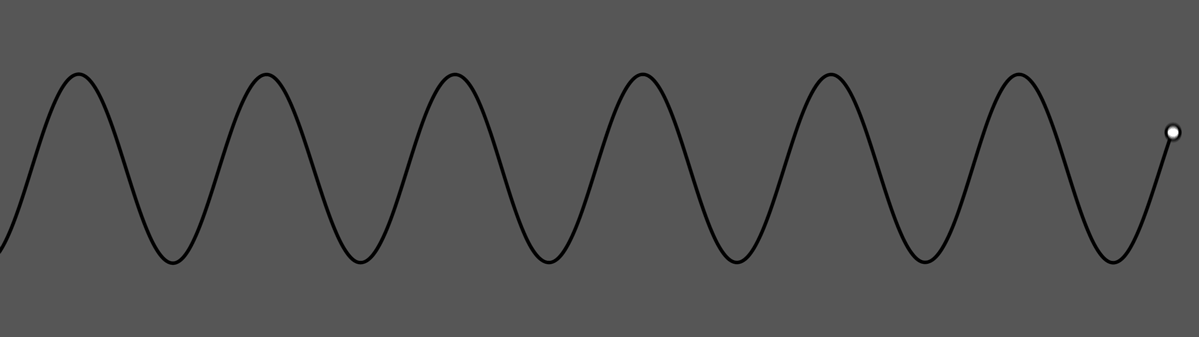Math Modifier

# Math Modifier

Generates modifier values from mathematical functions.## Method #

This node generates modifier values on the basis of mathematical pattern functions, from smooth sine waves to noise. A speed value of “1” equals one whole cycle taking 1 second, or 1 Hz if you will. The exception is the various noise functions that generate random values for every frame.

The node provides a preview of the pattern value that will be generated with a tracer line showing the place of the play head.

The output is a numeric value that can be further processed with other modifiers, or directly connected to a parameter in another node, using the operation method selected.

## Parameters

These properties control the core behaviours of the node.

ParameterDetails
Current ValueShows the current value of the effect. Cannot be directly changed.
Math FunctionThe mathematical function to be used by the modifier.
• Sine : A simple sine wave. Value = 1 equals 1 second per cycle.
• Cosine : A simple cosine wave. Value = 1 equals 1 second per cycle.
• Interpolated Noise : Random noise is generated with values and smoothly interpolated.
• Square : Jumps between 0 and 1 at a constant pace. Value = 1 equals 1 second per cycle.
• Square - Inverted : Jumps between 0 and 1 at a constant pace, at opposite timing to square. Value = 1 equals 1 second per cycle.
• Random Noise : Random values are generated at regular intervals with no interpolation between values.
• Perlin Noise : Noise is generated based on a gradient function, leading to smoother looking noise.
• Saw : Value Rises consistently, before sharply decreasing. Value = 1 equals 1 second per cycle.
• Triangle : Value rises then decreases at the same consistent rate. Value = 1 equals 1 second per cycle.
Blend AmountControl how much the modifier affects the values its input into.
ScaleAdjust the scale/range of the modifier.
OffsetApplies an offset (post scale) to the calculated value
SpeedThe speed at which the value moves through the generated pattern.
Time OffsetOffsets the time the value is modified from the time the waveform is played.
Time ModeHow the modifier updates with relation to the time code.
• Locked To Timecode : The modifier values are locked to the time code and the same time will always yield the same numerical value.
• Running / Looping : The modifier values are disconnected from the time code and will loop seamlessly at the end of the layer. This also means that animated speed values will change smoothly.
OperationThe operation to be performed on the targeted parameter.